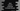# Java program to convert a decimal to binary format for a float or double value## Java program to convert a decimal to binary format for a float or double value:

In this post, we will write one Java program that will convert one decimal value to binary format. The value will be a floating point variable or double variable, i.e. it will have both integral and fractional parts.

For example, for the number 1.23, 1 is the integral part and .23 is the fractional part.

If we are converting 1.23 to binary, we have to convert both 1 and .23.

## How to convert floating numbers to binary numbers:

To convert a floating number to binary, we have to convert both integral and fractional parts.

### Convert the integral part to binary:

Follow the below steps to convert the integral part to binary:

• Find the remainder if we divide the number by 2. Save it in an array.
• Divide the number by 2 and set this value as the new value for the number.
• Repeat it until the number is greater than 2.
• Once done, print all remainders in reverse order.

### Convert the fractional part to binary:

Follow the below steps to convert the fractional part to binary:

• Multiply the fractional part by 2. Save the value before the decimal point.
• Set the fractional part of the new number and set it as the new number.
• Repeat these steps until it becomes 1.0.
• Print these values in the same order.

Let me show you with an example:

For the number 4.25, we need to find the binary for 4 and .25.

#### 4 to binary:

[current value] [remainder for divide by 2] [quotient for divide by 2]
4 0 2
2 0 1
1 1 0

If we take the remainders in reverse order, it will give us the binary for 4: 100

#### .25 to binary

[current value] [multiply by 2] [value before decimal]
.25 0.5 0
.5 1.0 1
0 stop stop

So, the binary for .25 is 01

Now, if we combine the binary results of 4 and .25, it will give us the binary for 4.25 i.e. 100.01 is the binary for 4.25

## Java Program:

Let’s write it down in a Java program:

``````import java.util.Scanner;

class MyClass {
private static String findBinary(double num) {
StringBuilder binaryBuilder = new StringBuilder();
int numIntegral = (int) num;
double numFractional = num - numIntegral;

// binary conversion for the integral part
while (numIntegral > 0) {
binaryBuilder.append(numIntegral % 2);
numIntegral /= 2;
}

binaryBuilder.reverse();

// binary conversion for the fractional part
if (numFractional != 0) {
binaryBuilder.append(".");

while (numFractional != 0) {
numFractional *= 2;
binaryBuilder.append((int) numFractional);
numFractional = numFractional - (int) numFractional;
}
}

return binaryBuilder.toString();
}

public static void main(String[] args) {
double num;
Scanner sc = new Scanner(System.in);

System.out.println("Enter a number: ");
num = sc.nextDouble();

System.out.println("Binary: " + findBinary(num));
}

}``````

In this program,

• findBinary method is used to convert a double to binary.
• This method breaks the double number to an integral and fractional parts and finds the binary values for each.
• It uses a StringBuilder and appends the binary value to this builder.
• First, it uses a while loop to find the binary of the integral part and appends that to the builder. Then it reverse the result.
• Next, it checks if there is any fractional value available or not. If yes, it appends a . and finds the binary for the fractional part, appends to the builder.
• Finally, it converts the StringBuilder to a string and returns that result.

### Sample output:

If you run this program, it will print output as like below:

``````Enter a number:
222.5
Binary: 11011110.1

Enter a number:
222
Binary: 11011110``````